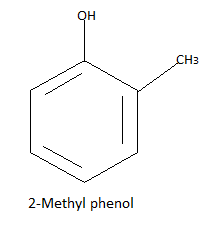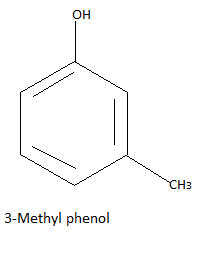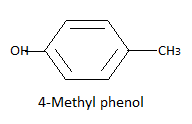Courses
Courses for Kids
Free study material
Free LIVE classes
MoreLIVE
Join Vedantu’s FREE Mastercalss

# Give the structure and IUPAC names of monohydric phenols of molecular formula, ${C_7}{H_8}O$.Verified
333.9k+ views
Hint: Phenol is formed when a hydrogen atom in a benzene ring is replaced by a hydroxyl group. $- OH$ group is called the hydroxyl group. Monohydric phenols are those which contain only one hydroxyl group.

Complete answer:In this question we have to find all the monohydric phenols of molecular formula ${C_7}{H_8}O$. We know phenols are aromatic compounds in which hydrogen is replaced by a hydroxyl group. In the benzene ring, there are only six carbons but in this question, seven carbons are given this means one hydrogen is replaced by a hydroxyl group and one hydrogen is replaced by some group containing carbon.
Due to extra carbon present in this formula, there will be an extra group other than the hydroxyl group (as we have to find monohydric phenols) and this extra group must contain carbon in it. And by interpreting the given formula there are three extra hydrogen atoms (one hydrogen from oh group four of benzene ring which is not replaced by any group so on subtracting these five hydrogen atoms from given eight hydrogen atoms we have extra three hydrogen atoms).
Now there is one carbon and three hydrogen atoms and one group to be formed so the only possibility is the methyl group formed by these four atoms. So we have three possible different structures with formula ${C_7}{H_8}O$ that is:Note:
Like monohydric phenols there exist dihydric phenols and trihydric phenols. In dihydric phenols, two hydrogen atoms are replaced by a hydroxyl group and in trihydric phenol three hydrogen atoms are replaced by a hydroxyl group. These compounds are the major source of phenolic pollution in rivers and streams.

Last updated date: 24th Sep 2023
Total views: 333.9k
Views today: 3.33k